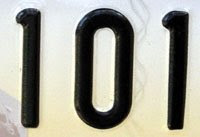## Tuesday, October 14, 2008

### 101

101 is a prime number. It is the smallest three-digit palindromic prime.

101 = 5! – 4! + 3! – 2! + 1!

101 is the sum of five consecutive primes: 13 + 17 + 19 + 23 + 29 = 101.

101 is the smallest odd prime that can be represented as the sum of a square and its reverse: 100 + 001 = 101.

A googol (10
100) contains 101 digits.John Nash's Princeton office number in the movie A Beautiful Mind was 101.

Source:
Prime Curios!